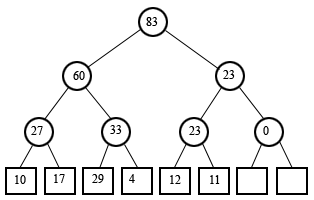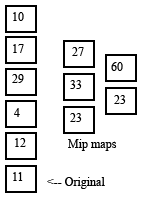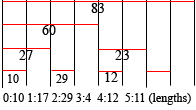# Web Rarely

Gentlemen, I want you to know that I am not always right, but I am never wrong. -Samuel Goldwyn

## a new data structure

2008-05-13

A couple months ago, when I was writing some code to implement a text editor, I was thinking about how I should design the data structure to map a character offset to a line number and column, and vice versa. I ended up designing what might be a novel data structure, along with several other data structures that other people have almost certainly also come up with. I benchmarked the three, and found that my data structure, while having some substantial benefits, also had a substantial drawback. I'm posting this partly because it's interesting, and partly in the hope that some smart person can comment on it.

The first data structure that popped into my mind was the simplest one -- an array, indexed by line number, storing the starting indices of the lines. The length of a line could be calculated by taking the difference between neighboring starting indices. With this array implementation, adding a new line to the end of the array and converting from line number to starting index are O(1) operations, but everything else is O(N). I quickly realized that it might be improved by storing the length of each line rather than the starting index of each line. Doing so means that changing the length of a line -- obviously a very common operation in a text editor -- becomes O(1), while converting a line number to its starting index becomes O(N). The array implementation has the benefit of being very simple, and I suspect many text editors use it.(the same thing in tree form)(mip maps)
I was thinking about how to speed up the operation of converting from line number to starting index and vice versa, since queries (for instance, during rendering) are probably more common than alterations, which usually only occur as fast as the user can press buttons. The first idea that came to mind was to "mip map" the arrays, with a higher level array storing the sum of several elements of the actual length array, allowing a fast search of the data. See the image to the right. From the look of it, I immediately thought "This looks like a binary tree. Why not use an actual binary tree for this?" The leaves would represent the individual lines, with the value stored at each leaf equal to that line's length. The value stored at each interior node is the combined length of all lines that are among its descendants. The value at the root, of course, is the total length of the document. The tree can even be packed into an array for efficient storage, in exactly the same way that a heap is. See the picture to the right for clarification. The rectangular nodes are leaves, representing the lengths of individual lines, and the circular nodes are interior nodes representing the sums of the line lengths of their descendants.

The upside to this data structure is that many operations that are O(N) in the array implementation are O(log N) in the tree implementation. Retrieving the length of a line remains O(1). However, some operations that are O(1) with the array, such as updating a line length, become O(log N) with the tree. Also, the space requirements are doubled compared to the array. Inserting, deleting, and adding lines are the slowest operations with this data structure. Although both the tree and the array have O(N) insertion and deletion time, the constant factors in the tree implementation make it take about six times longer. But I was confident that it was better than the array for large numbers of lines, because querying should be much more common than inserting and deleting lines.(a compressed version
of the above)
However, I was bothered by the increase in memory needed by the structure. The lengths of the lines were, in a sense, duplicated all the way up the tree. Of course it's this very duplication that allows the binary search to be as fast as it is, I thought, so perhaps it's a necessary evil. But a small voice in the back of my mind was saying "Maybe not!". When I listened more carefully, I saw that there was a way to remove some redundancy. If you consider the first two lines, with lengths 10 and 17, and their sum 27, they are related by the simple equation 10+17 = 27. So 10 could be calculated as 27-17, or 17 could be calculated as 27-10. One of the numbers is redundant. So if I stored the sum, I'd only need to store one of the lengths. The leaf nodes could store the length of every other line. Their parents would store the sum of every other pair of lines. And the parents of those sums would store the sum of every other group of 4 lines, and so on. So the data structure would only explicitly store half of the line lengths and sums, while the other half would be stored implicitly. See the image to the right. On the bottom row are the lengths of every other line (lines 0, 2, and 4). The next row above that contains the sums of lines 0+1, and of lines 4+5. That tree basically stores the same information as the tree above it, but more compactly. This brought the memory needs of the data structure back down. In fact, it needs the exact same amount of memory as the array, and yet it's something like a binary tree, so perhaps it could be queried more quickly than an array? If so, it might be the best of both worlds.(the spans of lines summed
for each tree node)
At this point I was intrigued, but I was doubtful that the data structure could be queried quickly enough to make it worthwhile. In fact, I wasn't even sure how to query it at all... let alone update it. That was the next thing to figure out. I began by querying the length of several lines by hand, and I noticed an interesting pattern. The number of nodes visited, and the pattern of the node accesses, was a function of the binary representation of the line number! That is, the bits that are set in the binary representation of the line number control which nodes need to be accessed. Look at the image to the right. The vertical black lines separate the line numbers and the horizontal red lines are the nodes in the tree. The extent of each red line indicates the line lengths that are summed to get the value stored at that node. Perhaps you notice the similarity between the pattern of extents of the red lines, and the set of integers that have a zero value for a given bit. For instance, the set of integers with the least significant bit (LSB) equal to zero are 0, 2, 4, 6, etc, which are the lines whose lengths stored in the bottom row of the tree. The set of integers with the next bit equal to zero are 0, 1, 4, 5, etc, matching the line sums 0+1 and 4+5 stored in the next level of the tree, and so on.

Given this revelation, I had an idea for how to query the data structure. Consider line number 3 (with zero-based counting). We know that the line has a length of 4. Here's how to calculate that from the tree. The line index 3, in binary, is 011. We start by positioning a node pointer to the leaf node nearest to the line we're looking up. The length of line 3 is not stored directly, but line 2 is, so we'll start there. We can simply mask off the LSB to get this index. In the tree above, you can see that line 2's length is 29. That's where the node pointer begins. We'll have a value that accumulates the length of the line, initialized to zero. If the LSB of the index is 1, we subtract the value stored at the node from the accumulator, shift the index to the right, move the node pointer up to the parent node, and repeat. If the LSB is 0, we add the value to the accumulator and the calculation is complete. Remember we have index 3, with binary representation 011. Since the LSB is 1, we subtract the current node value, 29, from the accumulator. The index is right-shifted, becoming 01, and the node pointer is moved to the parent, which stores the value 27. The LSB is still 1, so we subtract again. The accumulator becomes -56, the index becomes 0, and the node pointer moves to the node storing 60. The LSB is now equal to 0, and so we add 60 to the accumulator. -56 + 60 = 4, which is the length of the line. The offset of a line can be calculated very similarly, and simultaneously in fact. (You may notice a special case when retrieving the length of a line with an index that has all relevant bits set to 1, for instance the line at index 7 (binary 111) in a tree that can hold 8 lines. By the time the LSB of the index becomes zero, the node pointer has moved above the root of the tree. The elegant solution is to add a node above the root of the tree that stores the total length of the document (ie, a new root with only one child, which is the binary tree in the picture).

With that much worked out, it was also easy to figure out how to go the opposite way, converting a character index into a line number, by starting at the root of the tree and working downwards.

But I still didn't know how to update the data structure efficiently. Updating the length of a line seemed easy enough -- just propogate the length difference up the tree, adding it to the node value wherever a zero bit is found in the line index. But inserting and deleting lines efficiently has me stumped. Since the bottom row in the tree stores only the lengths of even-numbered lines, inserting or deleting a line causes all of the line numbers after it to be shifted by one. Even-numbered lines become odd-numbered, and vice versa. Since the lengths of odd-numbered lines are not stored directly in the tree, all of the new values for the leaf nodes after the insertion point would have to be recalculated. In my implementation, they are recalculated using the algorithm given above to retrieve a line length. This makes line insertion and deletion take O(N log N) time. It may be possible to recalculate them incrementally, but thinking about it made my brain hurt. Can you think of a faster way to insert or delete a line?

Complete source code and benchmarks for the data structures discussed are included in this source file. On the upside, the fancy tree structure is a bit faster than the simple tree structure at querying line information, and surprisingly, is orders of magnitude faster at adding lines to the end. And it only needs half as much space. On the downside, the benchmarks show the fancy tree structure taking 6 ms per line insertion/deletion compared to 0.31 ms for the simple tree structure, a factor of 19.4 difference, for trees holding 100,000 lines. Both the factor of the difference and the absolute times involved are substantially reduced for trees holding more typical (ie, smaller) numbers of lines, but even so, I was ultimately dissuaded from using my data structure because of its asymptotic complexity with regard to insertion and deletion.

23 or 33 2018-08-21 08:15AM
I think in the second and third picture (the binomial tree), it should be 23 and not 33 because the sum of the second pair (zero-based counting) is 23.
an anonymous w
RE: 23 or 33 2018-09-03 10:03AM
I believe you're right. Looks like a typo snuck in there. Now for the task of updating these 10-year-old graphs... Okay, I fixed them. Thanks!